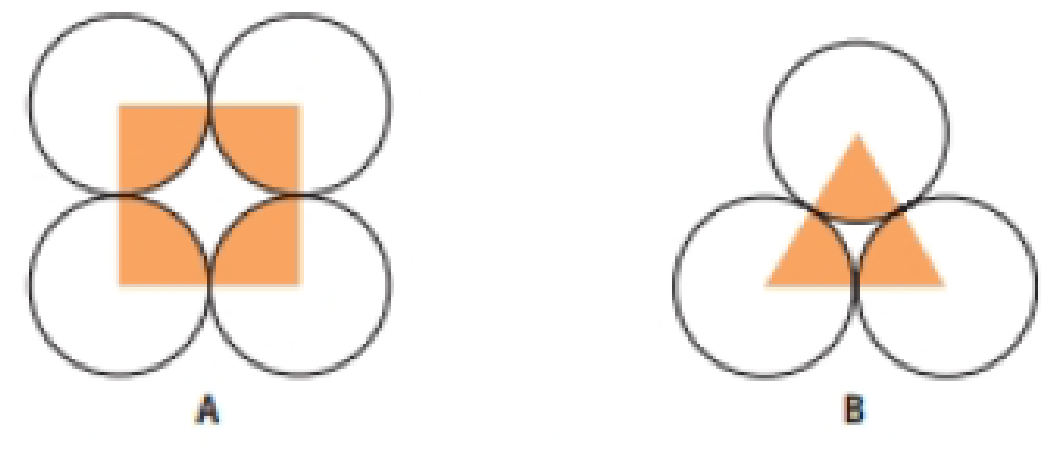# You can get some idea of how efficiently spherical atoms or ions are packed in a three-dimensional solid by seeing how well circular atoms pack in two dimensions. Using the drawings shown here, prove that B is a more efficient way to pack circular atoms than A. The unit cell of A contains portions of four circles and one hole. In B, packing coverage can be calculated by looking at a triangle that contains portions of three circles and one hole. Show that A fills about 80% of the available space, whereas B fills closer to 90% of the available space.### Chemistry & Chemical Reactivity

9th Edition
John C. Kotz + 3 others
Publisher: Cengage Learning
ISBN: 9781133949640

#### Solutions

Chapter
Section### Chemistry & Chemical Reactivity

9th Edition
John C. Kotz + 3 others
Publisher: Cengage Learning
ISBN: 9781133949640
Chapter 12, Problem 45GQ
Textbook Problem
1 views

## You can get some idea of how efficiently spherical atoms or ions are packed in a three-dimensional solid by seeing how well circular atoms pack in two dimensions. Using the drawings shown here, prove that B is a more efficient way to pack circular atoms than A. The unit cell of A contains portions of four circles and one hole. In B, packing coverage can be calculated by looking at a triangle that contains portions of three circles and one hole. Show that A fills about 80% of the available space, whereas B fills closer to 90% of the available space.Interpretation Introduction

Interpretation:

From the picture, picture B is more efficiency than picture A and diagram B is leads to the surface coverage of 78.5% has to be proved.

Concept introduction:

The area of the triangle is calculated by using following formula,

areaoftriangle=1/2×base×height

### Explanation of Solution

• Diagram A is leads to the surface coverage of 78.5% which is shown below,

=areacoveredbythecircletotalarea=areaofonecircle(2rcircle)2=Πr24r2=0.785=78.5%

• Diagram B is leads to the surface coverage of 78.5% which is shown below,

areaoftriangle=1/2×base×heightareaoftriangle=12×2r×3rareacovered

### Still sussing out bartleby?

Check out a sample textbook solution.

See a sample solution

#### The Solution to Your Study Problems

Bartleby provides explanations to thousands of textbook problems written by our experts, many with advanced degrees!

Get Started

Find more solutions based on key concepts
The top food sources of zinc include a. grapes. b. unleavened bread. c. shellfish. d. potato.

Nutrition: Concepts and Controversies - Standalone book (MindTap Course List)

What are some functions of skin?

Human Biology (MindTap Course List)

In what ways is the celestial sphere a scientific model?

Horizons: Exploring the Universe (MindTap Course List)

How can you determine that the speed of sound is the same for all frequencies by listening to a band orchestra?

Physics for Scientists and Engineers, Technology Update (No access codes included)